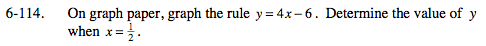### Home > MC2 > Chapter 6 > Lesson 6.2.5 > Problem6-114

6-114.Make a table of x-values and their corresponding y-values.
(To find the y-coordinate of an x-value, substitute the x-value
into the expression and solve.)

Plot these points onto your graph.(For this sample graph, we will only
plot 3 points.)

Draw a straight line that intersects all the points
plotted with arrows at the end to show that the
rule continues.

Estimate the y-value when the graph intersects x = 0.5.

Solve the problem using the eTool below.
Click the link at right for the full version of the eTool: MC2 6-114 HW eTool